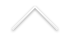Título: Phase transition for percolation on randomly stretched lattices

Palestrante: Augusto Q. Teixeira (IMPA)
Data: 03/05/2021
Horário: 15:00h
Local: Transmissão online.

Confira AQUI o link para a transmissão.

Resumo: In this talk we study the existence/absence of phase transitions for Bernoulli percolation on a class of random planar graphs. More precisely, the graphs we consider have vertex sets given by Z^2 and we start by adding all horizontal edges connecting nearest neighbor vertices. This gives us a disconnected graph, composed of infinitely many copies of Z, with the trivial behavior p_c(Z) = 1. We now add to G vertical lines of edges at {X_i}xZ, where the points X_i are given by an i.i.d. integer-valued renewal process with inter arrivals distributed as T. This graph G now looks like a randomly stretched version of the nearest neighbor graph on Z^2. In this talk we show an interesting phenomenon relating the existence of phase transition for percolation on G with the moments of the variable T. Namely, if E(T^{1+eps}) is finite, then G almost surely features a non-trivial phase transition. While if E(T^{1-eps}) is infinite, then p_c(G) = 1. This is a joint work with Hilário, Sá and Sanchis.